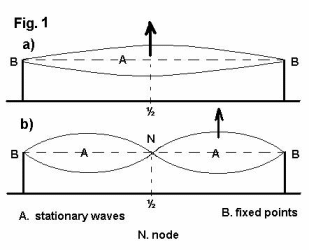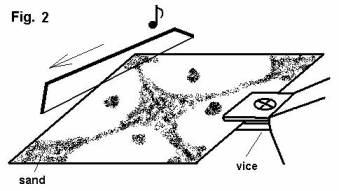THE VIBRATORY MOVEMENTS OF THE UNIVERSE
Just like the esoteric Eastern tradition of spirituality, modern physics does not conceive matter as being passive, inert, static, but as being in a continuous vibratory movement.

The rhythms of these movements are determined, among other things, by the atomic, nuclear and molecular structures. Therefore both science and spirituality converge toward the idea that the Universe is dynamic, being in a continuous movement of oscillation.

Nature is not in a static, but in a dynamic equilibrium. Here is what a Taoist text says about this matter:

“The silence in silence is not the true Silence. Only when there is calmness in movement, the spiritual rhythm [vibration] which penetrates the Sky and the Earth is born.”

Therefore everything is vibration. Here is what the scientists say about the subject:

“Everything is vibration. Everything is movement. The Void [Primordial Substratum] fluctuates, according to certain laws, between Existence and Non-existence. The quanta [a small, indivisible unit of energy] born as a result of the vibratory movement of the void, are ‘virtual’, i.e. they do not materialize into real particles that can be observed. They need a certain mass and energy in order to have material existence.”The whole Universe is made of lots of things and phenomena, from the smallest atoms to the greatest galaxies. Their common element is that they are all moving. WHY everything is moving is a question to which modern science has no answer.

Modern science has however made some progress in explaining HOW things move. Modern (quantum) physics states the existence of FOUR FORCES (assuming as a hypothesis the necessary existence of a FIFTH FORCE which unifies the first four).

This theory is a rediscovery of the eastern theory of the five tattva-s (or subtle principles – ‘earth’, ‘water’, ‘fire’, ‘air’, ‘ether’). According to physics, the four forces who act in the Universe are: the ‘strong’ and the ‘weak’ forces (who keep atoms together), the force of gravity (who describes the movement of bigger material bodies) and the electromagnetic force (such as light). All these four forces have something in common: they all move in ‘waves’.

Therefore the waves represent the basic movement of the Universe. Even though this subject may seem complicated, its basic principles are very simple. Once these principles are clearly understood, we can make progress in understanding the physical characteristics of resonance and harmony.A WAVE – ‘SOMETHING’ THAT MOVES
The propagation of light, for example, is sometimes explained as a flux of particles (photons – a quantum of electromagnetic energy) moving through space. But certain experiments produced results that cannot be explained if we consider that light is just a flux of particles. The fundamental element here is that even in these cases light nevertheless moves through space.

Searching for a more comprehensive model, at least in the case of light propagation, we can ask ourselves if there is also something else, besides particles, who can move from one place to another. The answer is yes, there is. If we throw a stone into a pond, we can see it: A WAVE.

As it was said above, just like in the Eastern doctrines, in modern physics there are already theoretical models that show that the entire Universe has vibration as its essential nature and that a certain modulated vibration can describe (build) a whole Universe.

For understanding easier this principle, let’s analize a one-dimensional vibration. Let’s suppose that we have a chord placed between two fixed points (fig.1a).

If we stretch the chord at its middle point and then let it go, the chord will start to oscillate and it will produce two symmetrical curves.

If we stretch the chord at a quarter of its length and then let it go, the chord will start to oscillate and it will produce the effect shown in fig.1b.This effect is called stationary (or standing) wave. Standing waves manifest only when the chord is acted upon at distances that divide its wavelength in integer parts. In fig. 1a, the length of the support is half a wavelength (therefore, nodes are identical with the two fixed points).

In fig. 1b the length of the support is one wavelength. We notice in fig.1b that the point N is static and remains so during the whole oscillation of the chord. This point is called a node. Nodes are points that do not move along a chord in stationary vibration.

Let’s suppose now that we have a thin metal plate that we fix on one side like in fig.2. We will spread on this sheet of metal a uniform and very thin layer of fine sand. Then, with a fiddlestick we touch one of the free sides until a sound is produced.As a result of the sound, the plate of metal will start to vibrate. We will notice then that the layer of sand will make certain shapes that are symmetrical. This shape is a result of the stationary waves created in the sheet of metal as a result of its vibration.

This is a bidimensional replica of the previous experiment. In this case, the sand accumulates in those areas where the metal plate does not vibrate (nodes) – in other words, the sand particles will accumulate in the points with the lowest energy.

Therefore, the stationary waves divide the metal plate in an integer number of wavelengths. The dimensions of the metal plate are important in creating these stationary waves and therefore the nodes with low energy.

When a certain structure enters in resonance (which means that at that time that structure vibrates on a certain frequency that is specific to that structure only and which can be maintained with least effort) this process of resonance will immediately produce stationary waves.